# Systems of equations solving systems of linear equations

## The concept of the system and its rozvytku

Definition: If the task is to find all common solutions of two (or more) equations with one or more variables, then we say that it is necessary razvesti system of equations.

Definition: Rozvyazka system — the value of a variable or such an ordered set of values smya that satisfies all the equations of the system, i.e. rozvyazka system of two or more equations with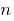unknown call such an ordered set a lotof numbers, after the substitution of which into the system is unknown, all the equations become true numerical equality.

Definition: Razvesti the system of equations is to find all its interchanges , or to prove that they are not.

If the system has no solution, then it is incompatible.

### Examples of systems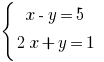— a system of two equations with two variables

Pair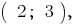that is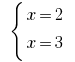a solution of the system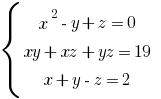— the system of three equations with three variables

Three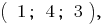that is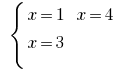one of rozvytku system

## Scheme the solution of systems of equations

### The graphical method

1. Perform equivalent transformations, so that it is convenient to graph the function. For example: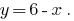2. Build graphics.
3. Find the point of intersection of the graphs. The coordinates of these points is rozvyazka this system of equations.

### The substitution method

1. One equation of the system we Express one variable via another, always choose a convenient variable. For example, from the equations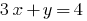we Express the variable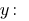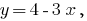and not Vice versa.
2. Substitute the value found into another equation of the system and obtain an equation with one variable.
4. The value found is substituted in the expressed equation and find the value of the second variable.

1. Urunov the coefficients of one variable by term multiplication of both equations on multipliers, selected accordingly.
2. Add (or subtract) pocino two equations of the system, thereby eliminating one variable.
4. Substitute the found value of the variable in any of the original equations.

## Examples of solving systems of equations

### Example 1

Rozwarte equation: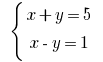Solutions:

Building graphics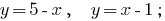Building graphics will see that the graphs intersect at the point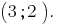Answer: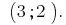### Example 2

Rozwarte equation: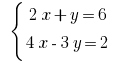Solutions:

From the first equation we Express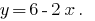And substitute the resulting expression into the second equation of the system: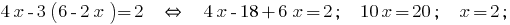The resulting valueis substituted into the expression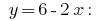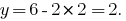Answer: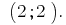### Example 3

Rozwarte equation: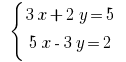Solutions:

Need to get rid of a variable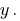poslano Multiply the first equation of system 3 and the second by 2.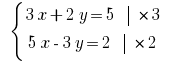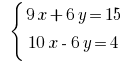Add pocino equation and get: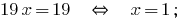Find the value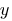of the first equation of the system:Answer: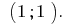Remark: the method add can be multiplied not only on positive numbers, and negative.

How rozwiazywanie the system of equations to solve only to You.

Tags:
Chapter:
Versions in other languages:
Share with friends: